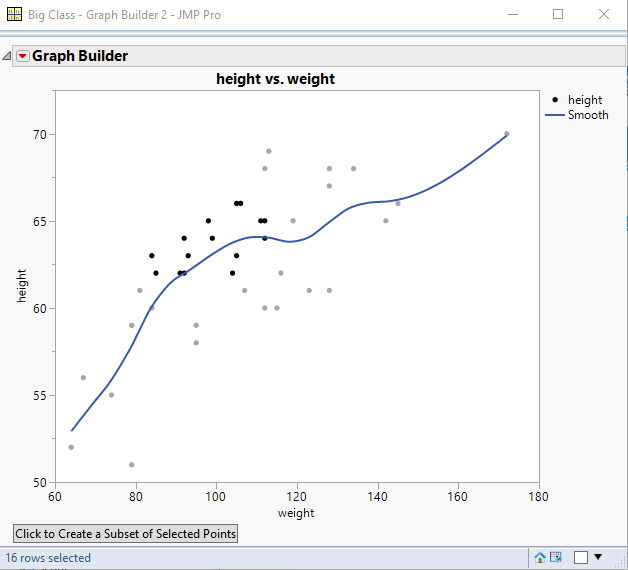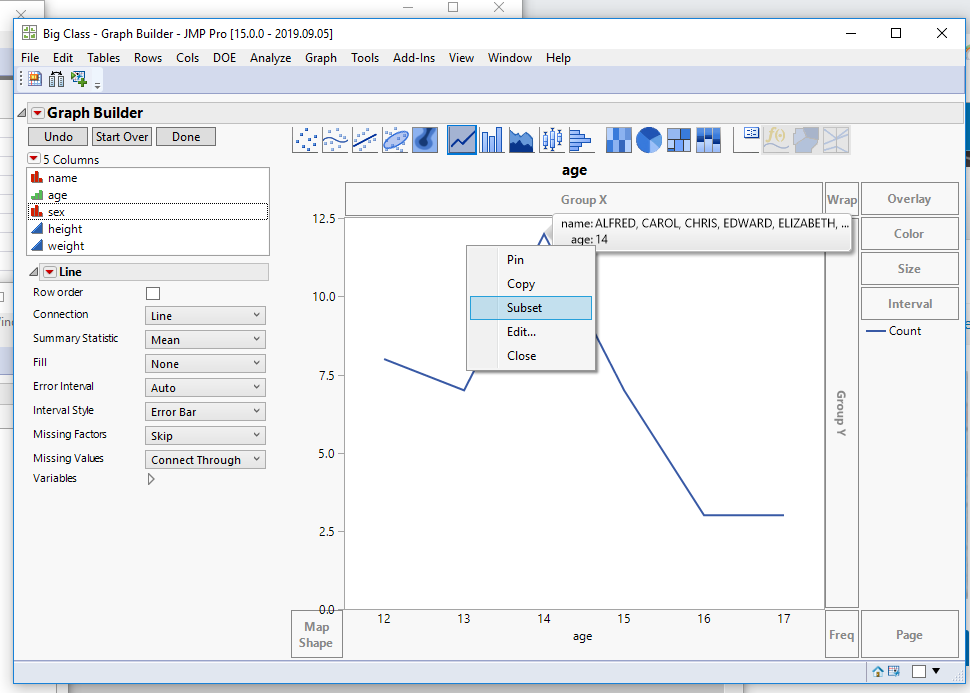Our World Statistics Day conversations have been a great reminder of how much statistics can inform our lives. Do you have an example of how statistics has made a difference in your life? Share your story with the Community!
Choose Language Hide Translation Bar
Highlighted

## Creating a Subset from an Interactive Graph?

Hello. I am trying to make an interactive graph to display data from a summary table. The goal is to create a line graph with points that can be clicked on to subset data from that particular point. For instance, in the script below, I have a graph of a summary table by age of the Big Class sample table. The user should be able to click on a point on the graph and have it automatically pull up a subset table containing all the data from that point.

The issue I am running in to is that a single click won't work because no data has been selected. The user must double click on a point to select the data, then click again to run the subset expression. Is there a way to do this without having to triple click? Thank you.

``````dt = Open( "\$SAMPLE_DATA/Big Class.jmp" );

dtSummary = dt << Summary(
Group( :age ),
Freq( "None" ),
Weight( "None" ),
output table name( "dtSummary" )
);

gbExpr = Expr(
gb = Graph Builder(
Size( 528, 448 ),
Show Control Panel( 0 ),
Variables( X( :age ), Y( :N Rows ) ),
Elements( Points( X, Y, Legend( 3 ) ), Line( X, Y, Legend( 4 ) ) ),
SendToReport(
Dispatch(
{},
"400",
LegendBox,
{Legend Position( {3, [-1], 4, [-1]} ), Position( {-1, -1} )}
)
)
);
);

ExprA = Expr(
dtSubset = dt << Subset( Output Table( "Subset" ), Selected Rows( 1 ), Selected Columns( 0 ));

dtSummary << Clear Selected Rows;
);

GraphWindow = New Window( "Graph" );

GraphWindow << Append(
MouseBox(
gbExpr,
<<SetClickEnable( 1 ),
<<SetClick( Function( {this, clickpt, event}, If(N Rows(dt << Get Selected Rows) < 1,, If( event == "Pressed", Eval( Expr( ExprA ))))))
)
);``````
1 ACCEPTED SOLUTION

Accepted Solutions
Highlighted

## Re: Creating a Subset from an Interactive Graph?

While doing all of the work to create a script that uses Mouse Traps, I think you can accomplish what you want with a very simple script, if you are willing to use the default mouse actions to select the data points, and then to just click on an added button to make the selection``````Names Default To Here( 1 );
dt = Open( "\$SAMPLE_DATA/big class.jmp" );

gb = dt << Graph Builder(
Size( 528, 454 ),
Show Control Panel( 0 ),
Variables( X( :weight ), Y( :height ) ),
Elements( Points( X, Y, Legend( 6 ) ), Smoother( X, Y, Legend( 7 ) ) )
);

subsetButton = Button Box( "Click to Create a Subset of Selected Points",
If( N Rows( dt << get selected rows ) > 0,
dt << subset( selected rows( 1 ), selected columns( 0 ) )
)
);

Report( gb )["Graph Builder"] << append( subsetButton );``````
Jim
4 REPLIES 4
Highlighted

## Re: Creating a Subset from an Interactive Graph?

While doing all of the work to create a script that uses Mouse Traps, I think you can accomplish what you want with a very simple script, if you are willing to use the default mouse actions to select the data points, and then to just click on an added button to make the selection``````Names Default To Here( 1 );
dt = Open( "\$SAMPLE_DATA/big class.jmp" );

gb = dt << Graph Builder(
Size( 528, 454 ),
Show Control Panel( 0 ),
Variables( X( :weight ), Y( :height ) ),
Elements( Points( X, Y, Legend( 6 ) ), Smoother( X, Y, Legend( 7 ) ) )
);

subsetButton = Button Box( "Click to Create a Subset of Selected Points",
If( N Rows( dt << get selected rows ) > 0,
dt << subset( selected rows( 1 ), selected columns( 0 ) )
)
);

Report( gb )["Graph Builder"] << append( subsetButton );``````
Jim
Highlighted

## Re: Creating a Subset from an Interactive Graph?

Perfect. Very helpful. Thank you very much.

Highlighted

## Re: Creating a Subset from an Interactive Graph?

An alternative to a Mouse Box is to apply a Row State Handler to the data table. It takes one click to create the subset (which can be annoying if trying to select multiple points by draging as a lot of unintended subsets may be created).

``````dt = Open("\$SAMPLE_DATA/Big Class.jmp");

dtSummary = dt << Summary(Group(:age));

gbExpr = Expr(
gb = Graph Builder(
Show Control Panel(0),
Variables(X(:age), Y(:N Rows)),
Elements(Points(X, Y, Legend(3)), Line(X, Y, Legend(4)))
)
);

ExprA = Expr(
dtSubset = dt << Subset(Selected Rows(1));
dtSummary << Clear Select;
);

f = Function({a}, ExprA);
rs = dt << make row state handler(f);
GraphWindow = New Window("Graph", gbExpr);
``````
Highlighted

## Re: Creating a Subset from an Interactive Graph?

In JMP 15.0, a Subset RMB menu option was added to hover labels that do exactly what you described.

Now all graphs come with this functionality built-in.

I just tried it and the results are exactly the same as when running your script.

Note that there is no need to create a separate summary table - the new feature "understands" the aggregations done by graphs like line charts, bar charts, etc.

In other words, the subset is based on a local data filter that is derived from the definition of the visual element under the pointer.

This technique also supports the creation of graphlets and their launching in new windows (aka drill-down).

For more details, check https://www.jmp.com/content/dam/jmp/documents/en/support/jmp15/using-jmp.pdf, page 512.Article Labels

There are no labels assigned to this post.